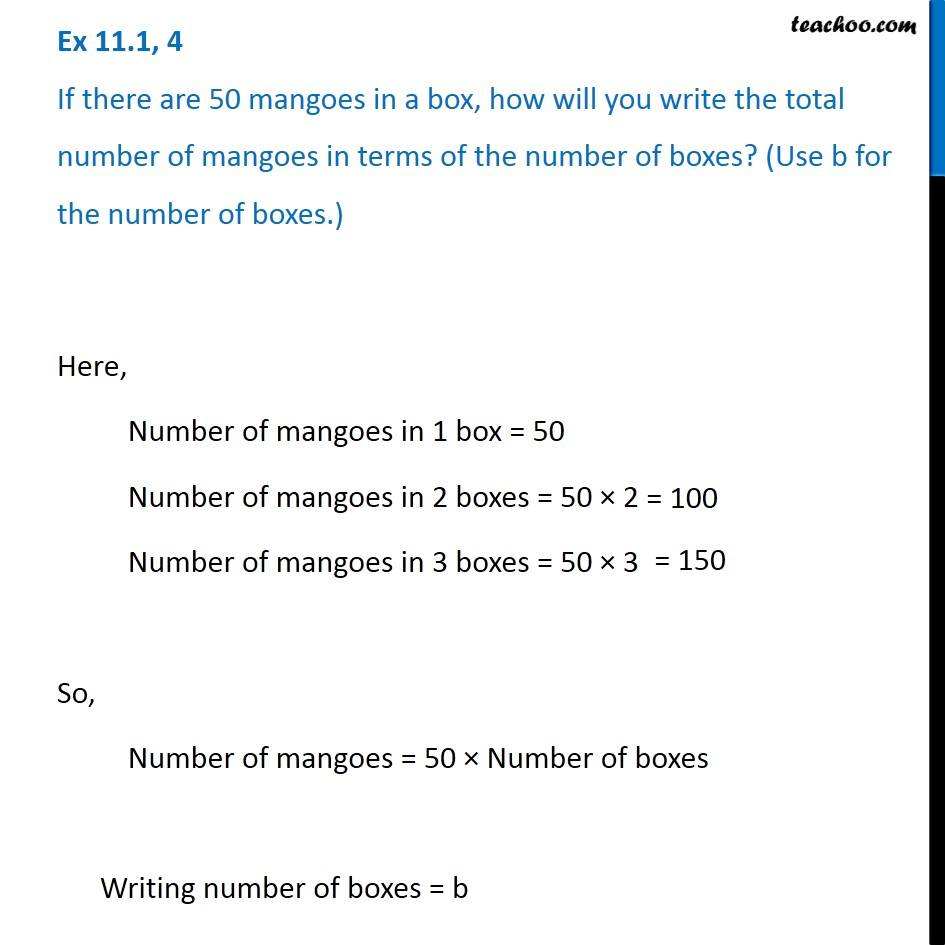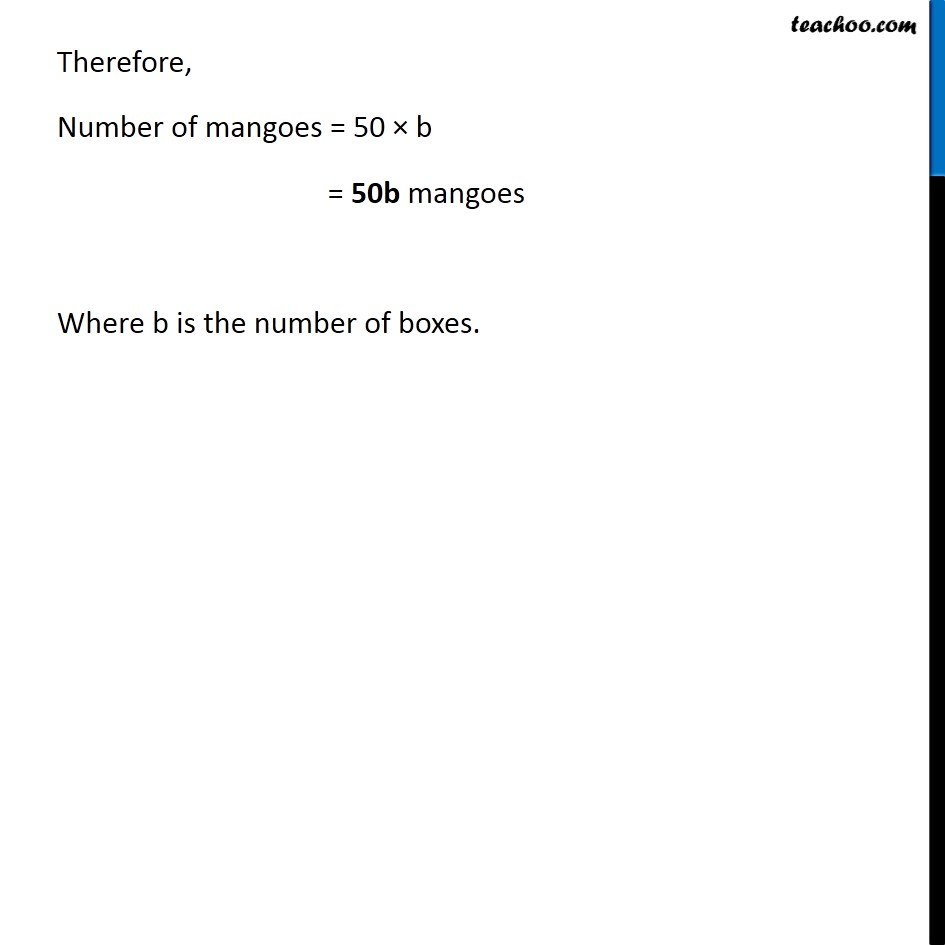Variable

Chapter 11 Class 6 Algebra
Concept wiseIntroducing your new favourite teacher - Teachoo Black, at only ₹83 per month

### Transcript

Ex 11.1, 4 If there are 50 mangoes in a box, how will you write the total number of mangoes in terms of the number of boxes? (Use b for the number of boxes.) Here, Number of mangoes in 1 box = 50 Number of mangoes in 2 boxes = 50 × 2 Number of mangoes in 3 boxes = 50 × 3 So, Number of mangoes = 50 × Number of boxes Writing number of boxes = b Therefore, Number of mangoes = 50 × b = 50b mangoes Where b is the number of boxes.